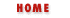#Chapter 4Expressions and Operators## 4.8 Assignment Operators

As we saw in the discussion of variables in Chapter 3, Variables and Data Types, = is used in JavaScript to assign a value to a variable. For example:

```i = 0
```
While you might not normally think of such a line of JavaScript as an expression that has a value and can be evaluated, it is in fact an expression, and technically speaking, = is an operator.

The = operator expects its left-hand operand to be a variable, or the element of an array or a property of an object, and expects its right-hand operand to be an arbitrary value of any type. The value of an assignment expression is the value of the right-hand operand. As a side effect, the = operator assigns the value on the right to the variable, element, or property on the left so that future uses of the variable, element, or property refer to the value.

Because = is defined as an operator, you can include it in more complex expressions. For example, you can assign and test a value in the same expression with code like this:

```(a = b) == 0
```
If you do this, be sure you are clear on the difference between the = and == operators!

The assignment operator has right-to-left associativity, which means that when multiple assignment operators appear in an expression, they are evaluated from right to left. This means that you can write code like the following to assign a single value to multiple variables:

```i = j = k = 0;
```

Remember that each assignment expression has a value that is the value of the right-hand side. So in the above code, the value of the first assignment (the rightmost one) becomes the right-hand side for the second assignment (the middle one) and this value becomes the right-hand side for the last (leftmost) assignment.

As we'll see in Chapter 7, Objects, you can use the Object.assign() method in Navigator 3.0 to override the behavior of the assignment operator.

### Assignment with Operation

Besides the normal = assignment operator, JavaScript also supports a number of other assignment operators that provide a shortcut by combining assignment with some other operation. For example, the += operator performs addition and assignment. The following expression:

```total += sales_tax
```
is equivalent to this one:

```total = total + sales_tax
```

As you might expect, the += operator works for numbers or strings. For numeric operands, it performs addition and assignment, and for string operands, it performs concatenation and assignment.

Similar operators include -=, *=, &=, and so on. Table 4.2 lists them all. In general, the expression:

```a op= b
```
where op is an operator, is equivalent to:

```a = a op b
```

Table 4.2: Assignment Operators
Operator Example Equivalent
+= a += b a = a + b
-= a -= b a = a - b
*= a *= b a = a * b
/= a /= b a = a / b
%= a %= b a = a % b
<<= a <<= b a = a << b
>>= a >>= b a = a >> b
>>>= a >>>= b a = a >>> b
&= a &= b a = a & b
|= a |= b a = a | b
^= a ^= b a = a ^ bBitwise OperatorsMiscellaneous Operators